## Homework 7

1. A particle is in the state. Find the expected values of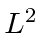,,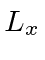, and.

2. A particle is in the state. If a measurement of thecomponent of angular momentum is made, what are the possilbe outcomes and what are the probabilities of each?

3. Calculate the matrix elementsand4. The Hamiltonian for a rotor with axial symmetry is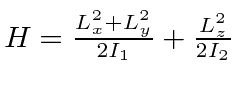where the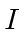are constant moments of inertia. Determine and plot the eigenvalues offor dumbbell-like case that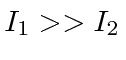.

5. Prove thatis only possible for.

6. Write the spherical harmonics for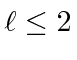in terms of the Cartesian coordinates,, and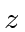.

7. A particle in a spherically symmetric potential has the wave-function. A measurement ofis made. What are the possible results and the probabilities of each? If the measurement ofyields, what are the possible measured values ofand what are the corresponding probabilities?

8. The deuteron, a bound state of a proton and neutron with, has a binding energy of -2.18 MeV. Assume that the potential is a spherical well with potential of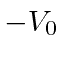forFermis and zero potential outside. Find the approximate value ofusing numerical techniques.

Jim Branson 2013-04-22